maths

Mensuration : Length, Area, and Volume

Welcome to the refreshingly new views to calculating perimeter, area, and volume of 2D and 3D shapes.

•  Length is Distance-Span, measured in reference span of to $1$$1$ unit long line

•  Area is Surface-Span, measured in reference to span of $1×1$$1 \times 1$ square

•  Volume is Space-Span, measured in reference to span of $1×1×1$$1 \times 1 \times 1$ cube

In this basic course, the following are covered.

•  Perimeter and area of simple 2D shapes

•  surface area and volume of simple 3D shapes

maths > mensuration-basics > basicmensu-measuring-standard

Introduction to Standards

This topic introduces the following

•  What are standard in measurement?

•  What are Absolute Standards?

•  What are Derived Standards?

Absolute Standards : An unit of measurement, widely accepted and used in reference to a standardized prototype.

Derived Standards : An unit of measurement that is defined using other absolute standards.

maths > mensuration-basics > basicmensu-measuring-length

Introduction to Measuring Length

This topic introduced measuring length as "distance-span". It is an absolute standard and measured in reference to a standard $1$$1$ unit.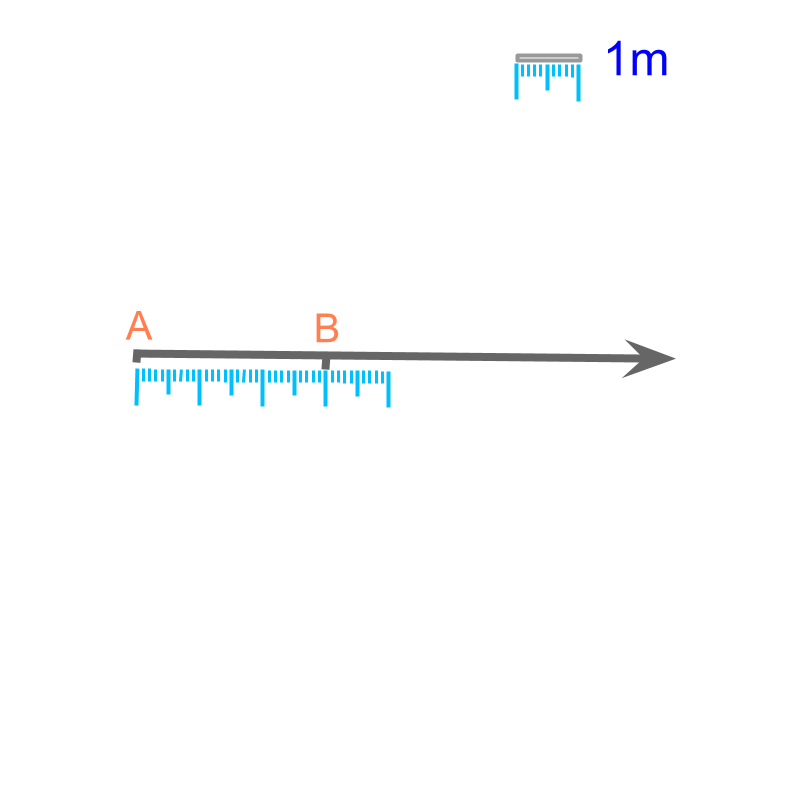Length : The distance-span between two points is the length. It is measured in meter or in one of its other forms. Length is specified as a number in reference to the reference-prototype-standard meter (or in one of other derived or similar forms).

maths > mensuration-basics > measuring-length-approximation

Accurate measure and Approximate measure

In this topic accuracy of measurement is explained.

Accuracy of Measurements : Measurement can be performed to a desired accuracy level.

When the accuracy is specified to a lower level, the measurement is approximate measurement.

maths > mensuration-basics > basicmensu-measuring-area

Introduction to Measuring Area

This topic introduced measuring area as "surface-span". It is a derived standard and measured in reference to a standard $1x1$$1 x 1$ square.

Area of a plane figure : The surface-span of a plane figure is the area of the surface. It is measured in square meter (or in one of other derived or similar forms).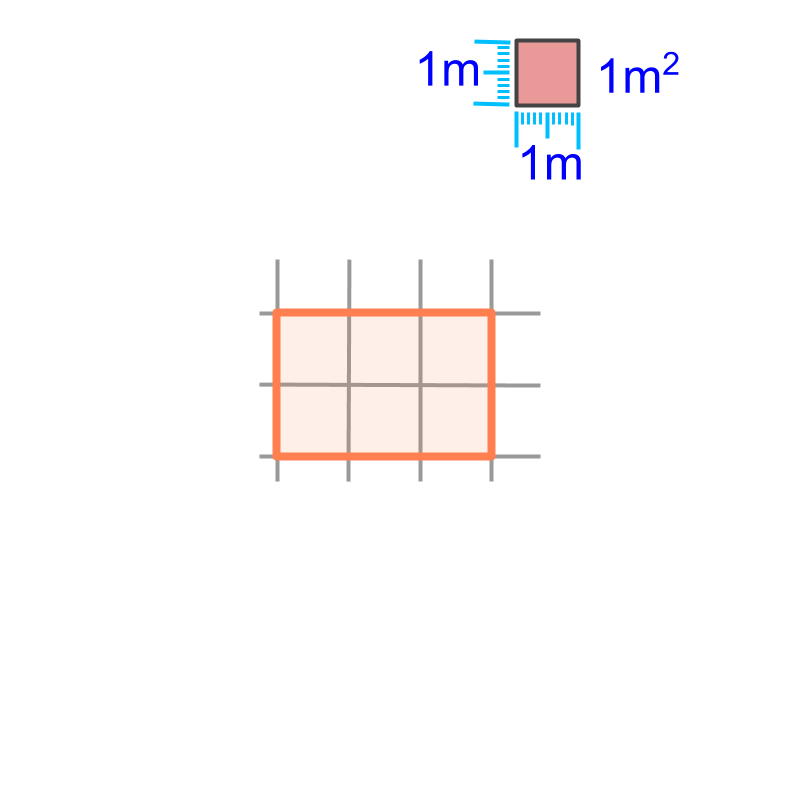Area is specified as a number in reference to the surface-span of a square of $1$$1$ meter side.

maths > mensuration-basics > basicmensu-measuring-volume

Introduction to Measuring Volume

This topic introduced measuring volume as "space-span". It is a derived standard and measured in reference to a standard $1×1×1$$1 \times 1 \times 1$ cube.

Volume of a solid : The space-span of a solid is the volume of the solid. It is measured in cubic meter (or in one of other derived or similar forms.)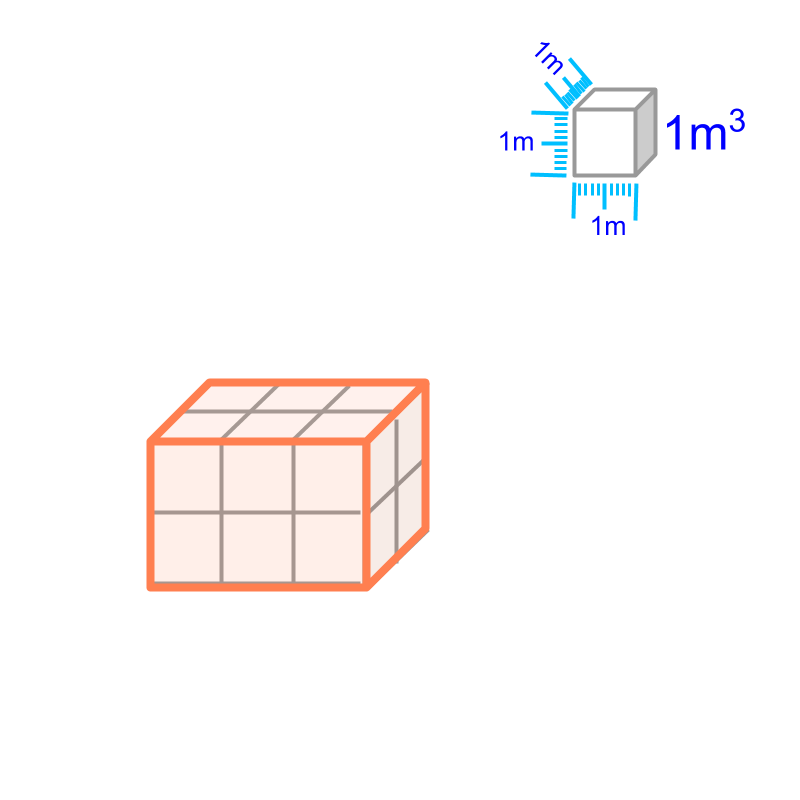Volume is specified as a number in reference to the space-span of a cube of $1$$1$ meter side.

maths > mensuration-basics > basicmensu-units-conversion

Conversion of Units of Measure

This topic provides a very brief overview of conversion of Units of Measure.

maths > mensuration-basics > basic-perimeter-polygons

Perimeter: Square, Rectangle, Triangle, Polygons

Finding perimeter of simple figures (squares, rectangle, triangle, polygon) are revised.

Perimeter :
Perimeter of a figure is the length of the line or curve forming the boundary of the figure.

Perimeter of square $=4×\phantom{\rule{1ex}{0ex}}\text{side}$$= 4 \times \textrm{s i \mathrm{de}}$

Perimeter of rectangle $=2×\phantom{\rule{1ex}{0ex}}\text{length + width}$$= 2 \times \textrm{\le n > h + w i \mathrm{dt} h}$

Perimeter of triangle $=\phantom{\rule{1ex}{0ex}}\text{sum of three sides}$$= \textrm{\sum o f t h r e e s i \mathrm{de} s}$

Perimeter of polygon $=\phantom{\rule{1ex}{0ex}}\text{sum of sides}$$= \textrm{\sum o f s i \mathrm{de} s}$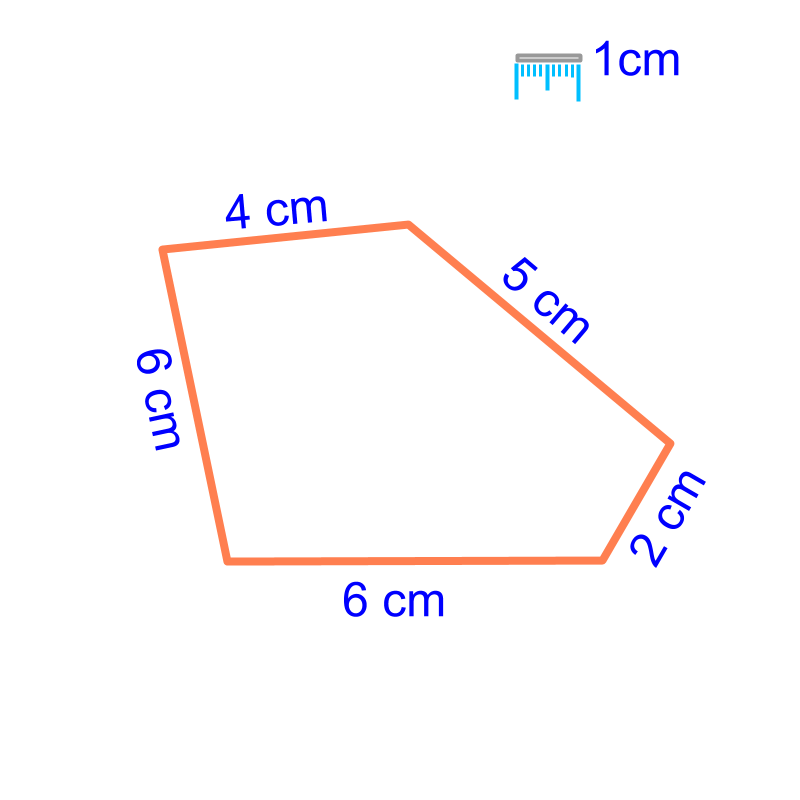maths > mensuration-basics > basic-area-square-rectangle

Area of Square and Rectangle

Area of a Square and a Rectangle :
Area of square $={\phantom{\rule{1ex}{0ex}}\text{side}}^{2}$$= {\textrm{s i \mathrm{de}}}^{2}$

Area of rectangle $=\phantom{\rule{1ex}{0ex}}\text{length}\phantom{\rule{1ex}{0ex}}×\phantom{\rule{1ex}{0ex}}\text{width}$$= \textrm{\le n > h} \times \textrm{w i \mathrm{dt} h}$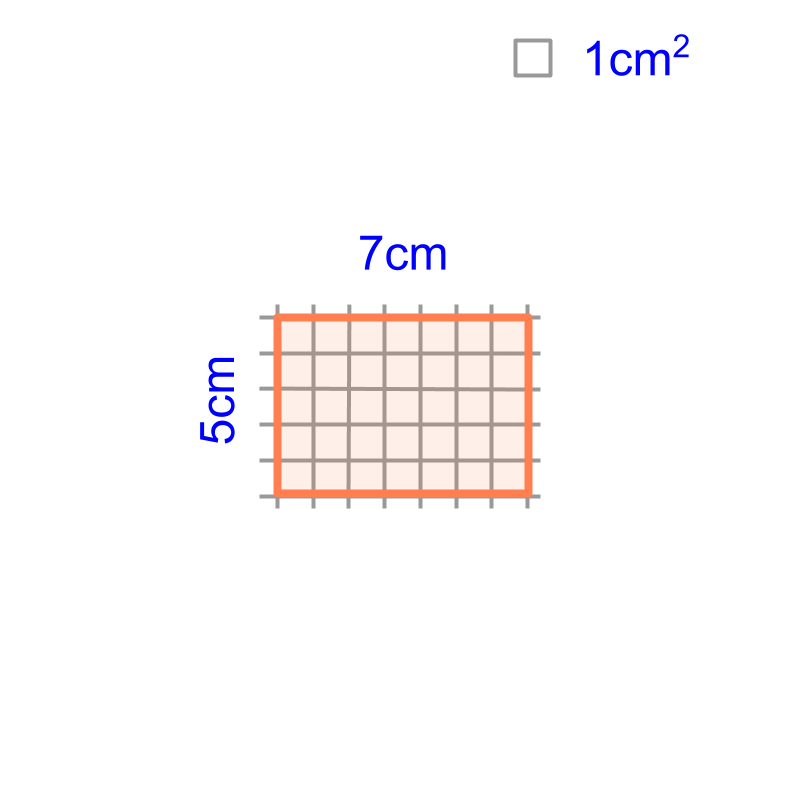maths > mensuration-basics > basic-area-triangle

Area of a Triangle

The area of a triangle is calculated based on geometrical properties. In this chapter, the different configurations of triangles are illustrated and a common formula is derived.

Area of a Triangle:
$\frac{1}{2}×\phantom{\rule{1ex}{0ex}}\text{base}\phantom{\rule{1ex}{0ex}}×\left(height\right)$$\frac{1}{2} \times \textrm{b a s e} \times \left(h e i g h t\right)$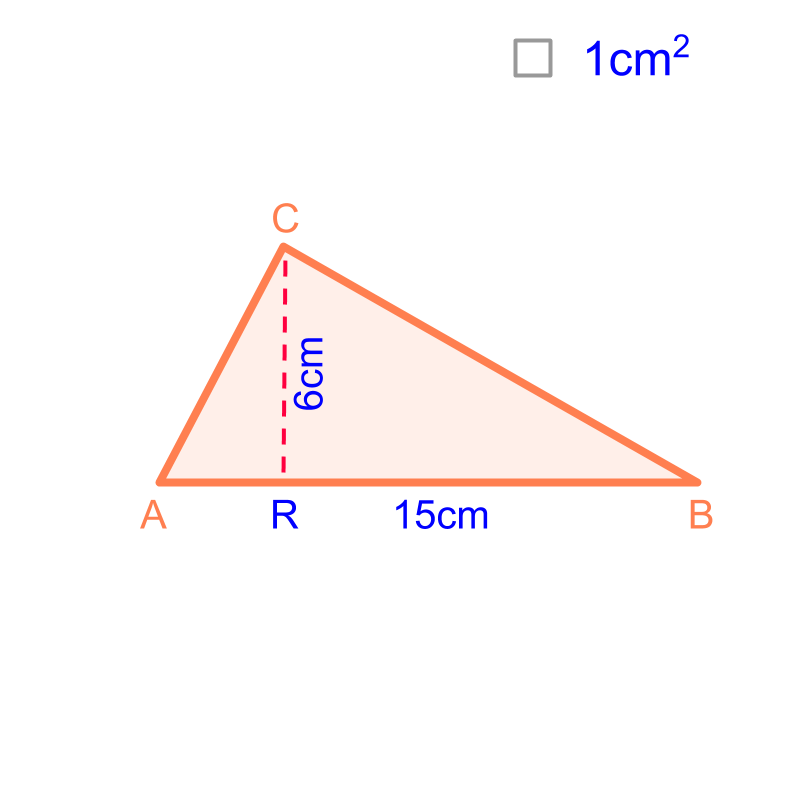maths > mensuration-basics > basic-area-polygons

Area of Polygons

Area of a Polygon : Consider a polygon to be combination of known geometrical forms, mostly triangles.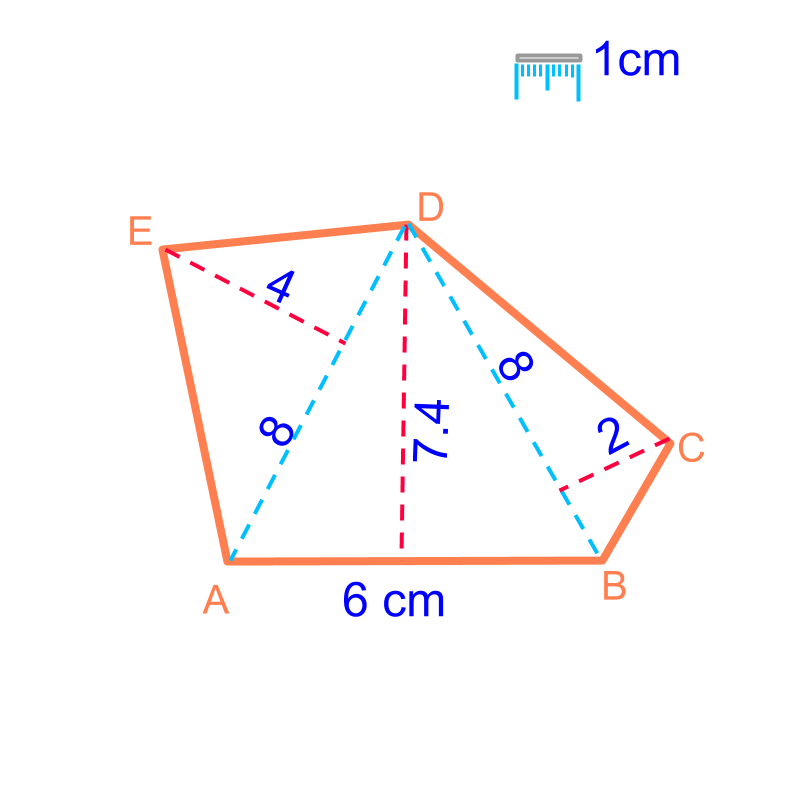The geometrical forms and the formula for area are:

Area of a triangle $=\frac{1}{2}×\phantom{\rule{1ex}{0ex}}\text{base}\phantom{\rule{1ex}{0ex}}×\phantom{\rule{1ex}{0ex}}\text{height}$$= \frac{1}{2} \times \textrm{b a s e} \times \textrm{h e i g h t}$

Area of a trapezium $=\frac{1}{2}×\phantom{\rule{1ex}{0ex}}\text{sum of bases}\phantom{\rule{1ex}{0ex}}×\phantom{\rule{1ex}{0ex}}\text{height}$$= \frac{1}{2} \times \textrm{\sum o f b a s e s} \times \textrm{h e i g h t}$

Area of a parallelogram $=\phantom{\rule{1ex}{0ex}}\text{base}\phantom{\rule{1ex}{0ex}}×\phantom{\rule{1ex}{0ex}}\text{height}$$= \textrm{b a s e} \times \textrm{h e i g h t}$

Area of a kite $=\frac{1}{2}×\phantom{\rule{1ex}{0ex}}\text{major-diagonal}\phantom{\rule{1ex}{0ex}}×\phantom{\rule{1ex}{0ex}}\text{d2}$$= \frac{1}{2} \times \textrm{m a j \mathmr{and} - \mathrm{di} a g o n a l} \times \textrm{d 2}$

maths > mensuration-basics > basic-perimeter-area-circle

Circumference and Area of a Circle

In this page, the formula to find circumference of a circle is introduced.

Circumference of a Circle: :
Circumference $=2\pi r$$= 2 \pi r$ and $=\pi d$$= \pi d$

$r$$r$ is the radius of the circle

$d$$d$ is the diameter of the circle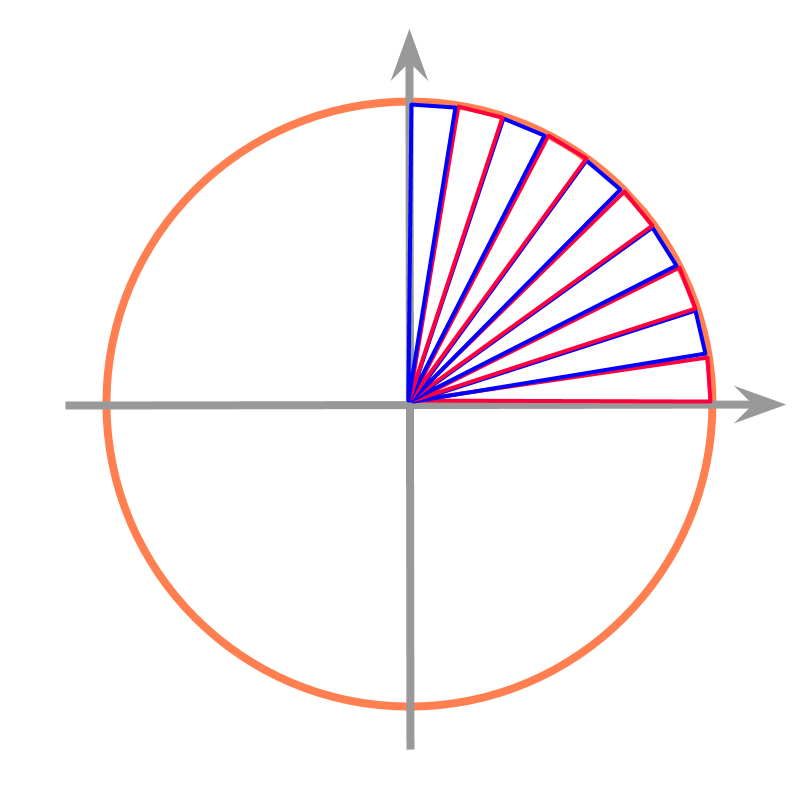Area of a Circle :
$=\pi {r}^{2}$$= \pi {r}^{2}$

$r$$r$ is the radius of the circle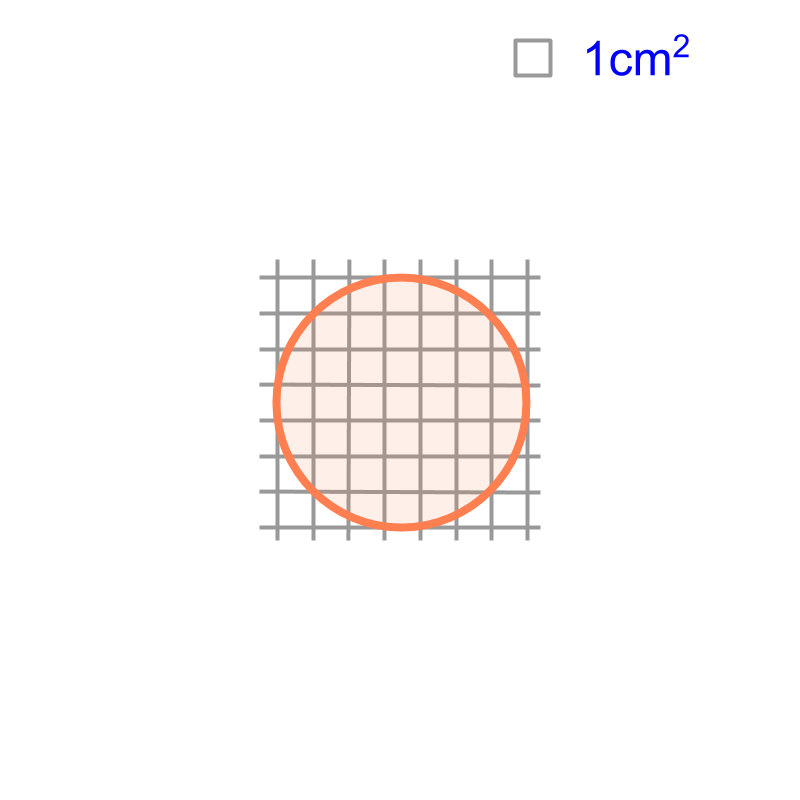Perimeter and Area of Various Quadrilaterals

Area of Some Quadrilaterals : Consider the polygon shapes as combination of triangles and find sum of area of the triangles.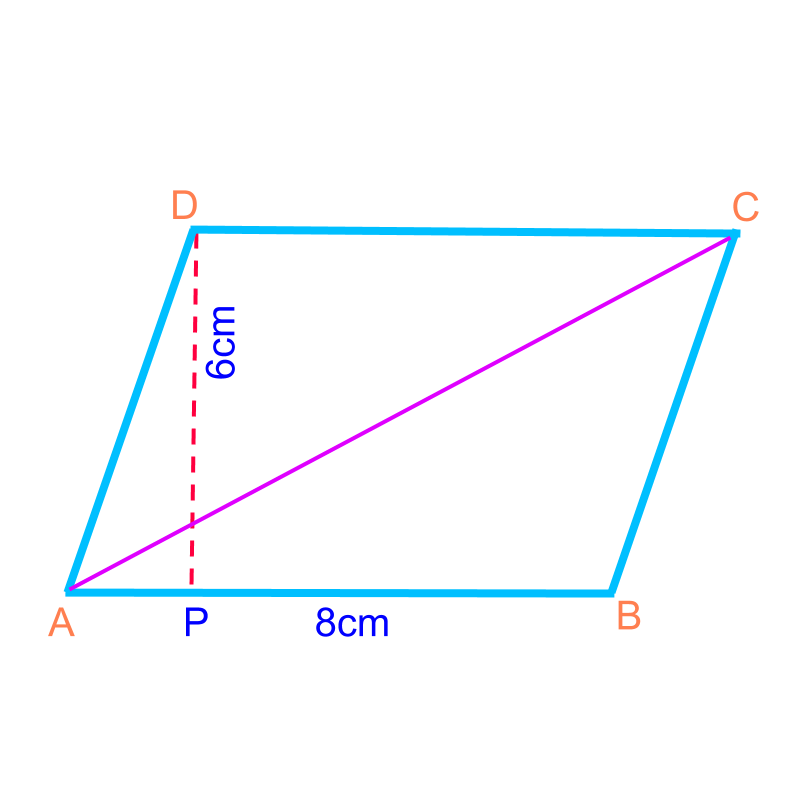$\phantom{\rule{1ex}{0ex}}\text{area of a parallelogram}\phantom{\rule{1ex}{0ex}}$$\textrm{a r e a o f a p a r a l \le \log r a m}$$=\phantom{\rule{1ex}{0ex}}\text{base}\phantom{\rule{1ex}{0ex}}×\phantom{\rule{1ex}{0ex}}\text{height}$$= \textrm{b a s e} \times \textrm{h e i g h t}$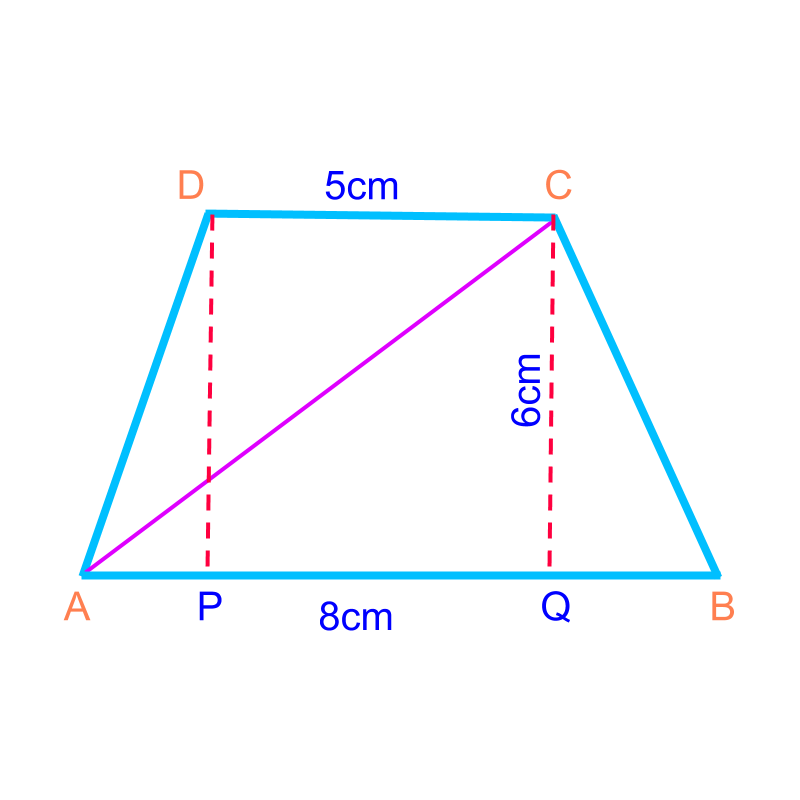$\phantom{\rule{1ex}{0ex}}\text{area of a trapezium}\phantom{\rule{1ex}{0ex}}$$\textrm{a r e a o f a t r a p e z i u m}$$=\frac{1}{2}×\phantom{\rule{1ex}{0ex}}\text{sum of bases}\phantom{\rule{1ex}{0ex}}×\phantom{\rule{1ex}{0ex}}\text{height}$$= \frac{1}{2} \times \textrm{\sum o f b a s e s} \times \textrm{h e i g h t}$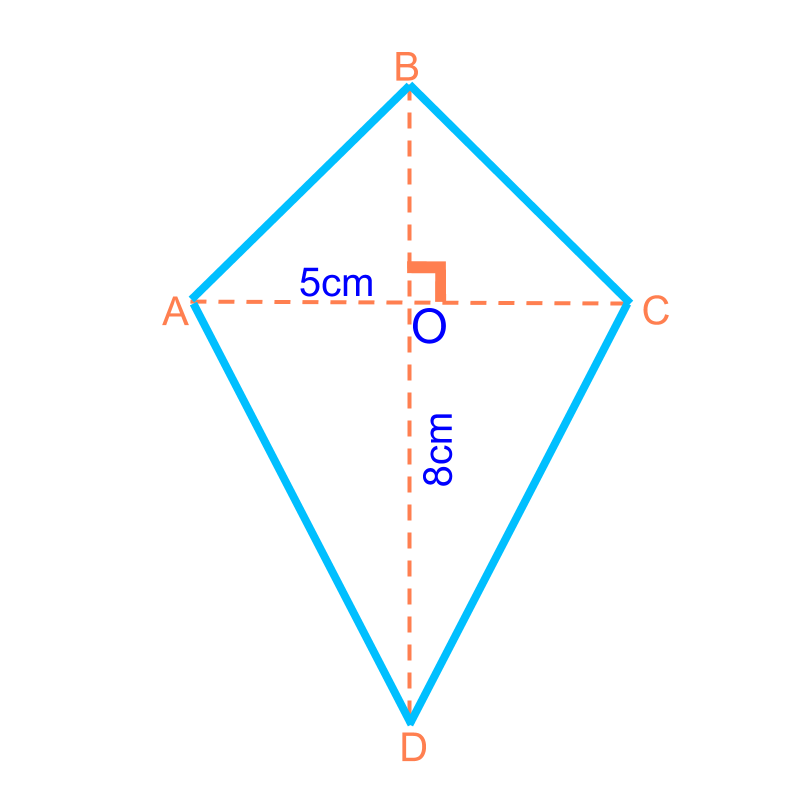$\phantom{\rule{1ex}{0ex}}\text{area of a kite}\phantom{\rule{1ex}{0ex}}$$\textrm{a r e a o f a k i t e}$$=\frac{1}{2}×\phantom{\rule{1ex}{0ex}}\text{product of the diagonals}$$= \frac{1}{2} \times \textrm{\prod u c t o f t h e \mathrm{di} a g o n a l s}$

maths > mensuration-basics > basic-surface-area-basic-shapes

Surface Area of Cube, Cuboid, Cylinder

In this page, Finding surface area of simple figures (cube, cuboid, and cylinder) are revised without much discussion.

Surface Area of Some Shapes: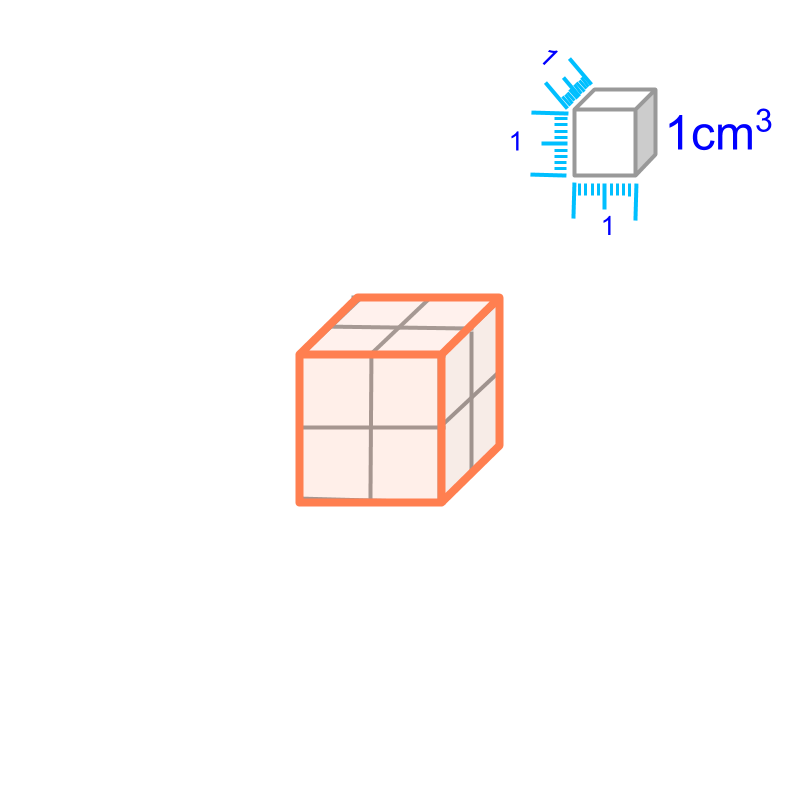Surface Area of cube $=6{q}^{2}$$= 6 {q}^{2}$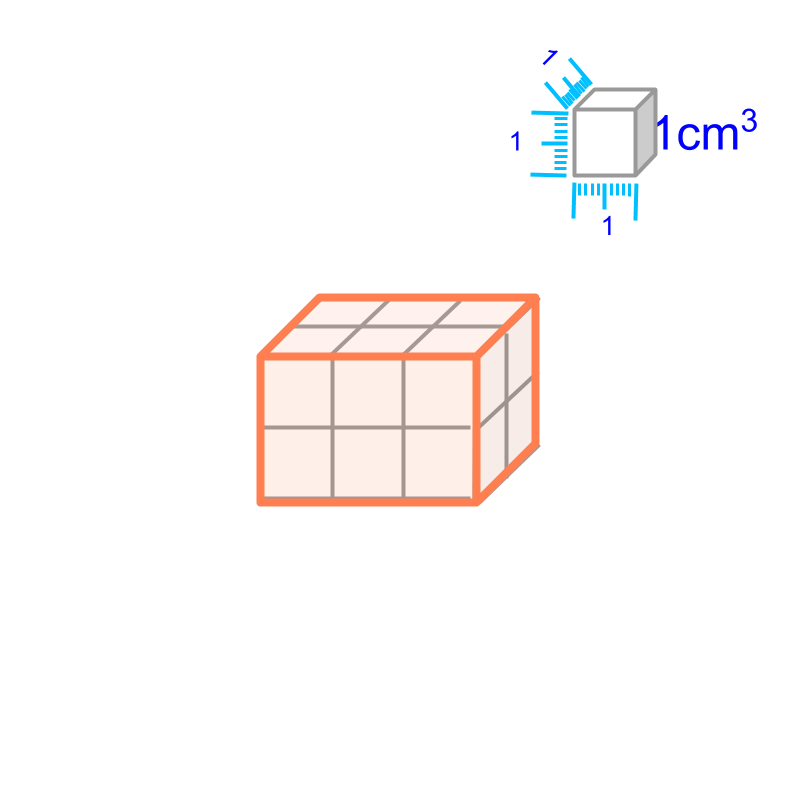Surface Area of cuboid $=2\left(lb+bh+hl\right)$$= 2 \left(l b + b h + h l\right)$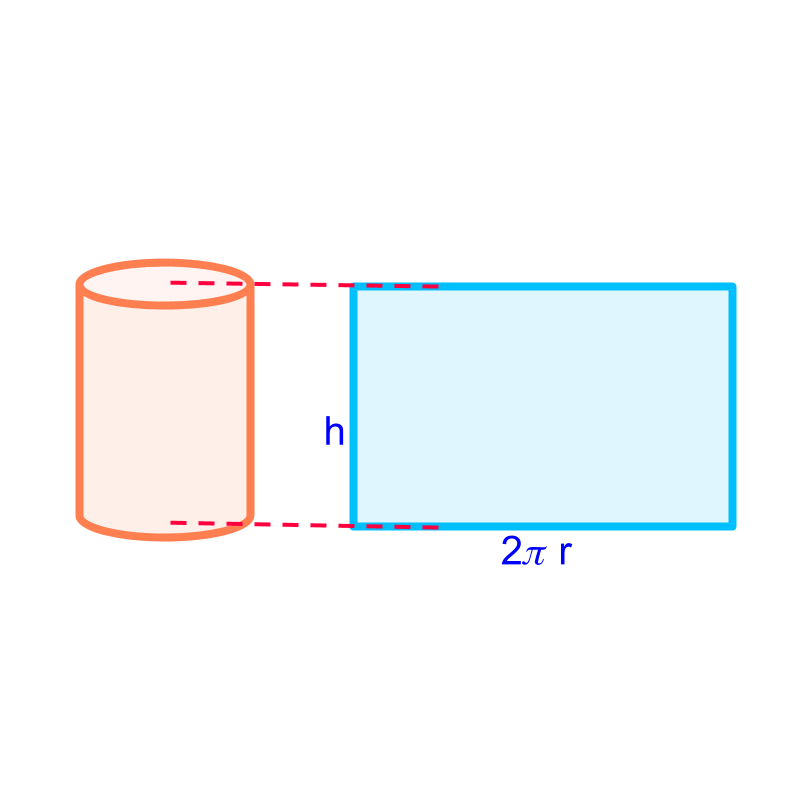Curved Surface Area of Cylinder $=2\pi rh$$= 2 \pi r h$

Surface Area of cylinder $=2\pi r\left(r+h\right)$$= 2 \pi r \left(r + h\right)$

maths > mensuration-basics > basic-volume-basic-shapes

Volume : Cube, Cuboid, Cylinder

In this page, Finding volume of simple figures (cube, cuboid, and cylinder) are revised without much discussion.

Volume of Some Solids :Volume of cube $={a}^{3}$$= {a}^{3}$Volume of cuboid $=lbh$$= l b h$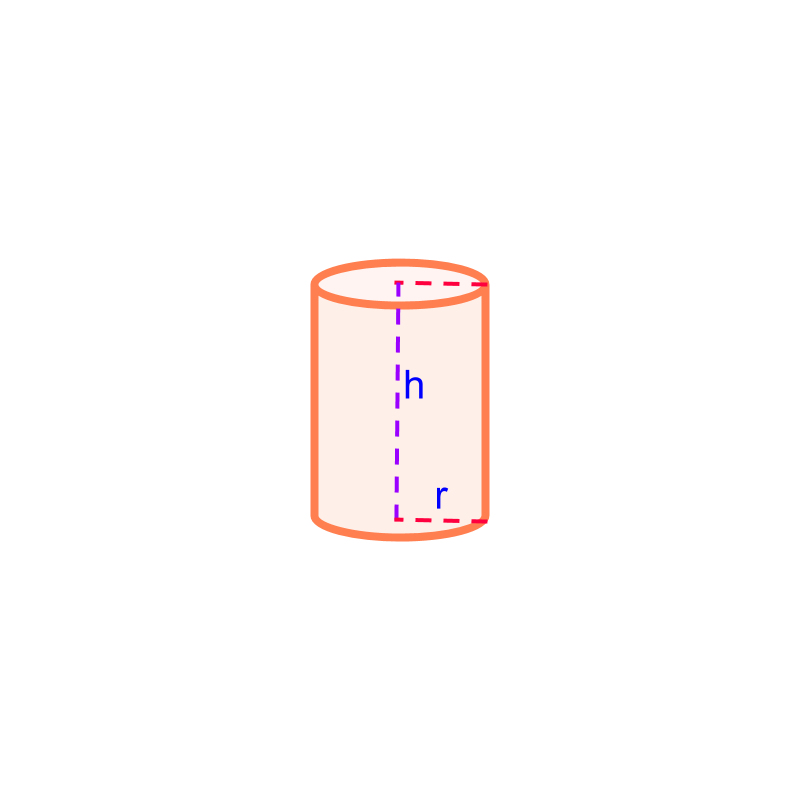Volume of cylinder $=\pi {r}^{2}h$$= \pi {r}^{2} h$